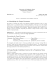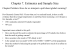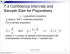# Objectives Qualls - 6. Confidence Interval of Population Proportion Lecture 6

## Transcription

Objectives Qualls - 6. Confidence Interval of Population Proportion Lecture 6
```Qualls - 6. Confidence Interval of Population Proportion
Objectives
Lecture 6
At the end of this section you should be able to answer
questions concerning point and interval estimates of
a population proportion, and determining the
requisite sample size for a given confidence level.
Confidence Intervals
and Sample Sizes
Part 2: Proportions
Specifically, you should understand:
• the difference between a point estimate and an
interval estimate
• how to calculate a confidence interval for a
population proportion
• how to determine the requisite sample size given a
desired margin of error and confidence level.
DePaul University
Bill Qualls
1
2
Point Estimate of a Population Proportion
• We used the following example when we introduced
the binomial distribution: Assume my free-throw
average is 40%. If I throw 3 free-throws, what is the
probability that I will miss all three? Hit 1? Hit 2? Hit
3?
Confidence Intervals
Population Proportion
• In our problems dealing with binomial probabilities,
we have been given p. But what if we would like to
estimate p with a known level of confidence?
3
4
Point Estimate of a Population Proportion
Point Estimate of a Population Variance
• A point estimate is a single value used to
• Given that the variance for a binomial
approximate a population parameter.
distribution is defined as σ²=npq, where q = 1-p,
and that p-hat is the best estimate for the population
• The best point estimate ("p-hat") of a population
parameter p, it follows then that the best estimate
proportion (p) is the sample proportion.
for the population variance is:
successes
pˆ =
trials
σˆ 2 = npˆ qˆ where qˆ = 1 − pˆ
• The use of a carat symbol (^) over a letter is read as
"hat", and indicates it is an estimated value.
5
Updated 3/15/2014
6
Qualls - 6. Confidence Interval of Population Proportion
Interval Estimates
The Problem with Point Estimates
• If I sink 4 free-throws out of 10, then my point
estimate for p is .4.
• We can, however, assign a level of confidence to an
interval estimate.
• Likewise, if I sink 40 free-throws out of 100, then my
point estimate for p is still .4.
• We would intuitively have more confidence in the
second statistic than in the first.
• If you were asked to come up with a 95% confidence
interval for the first case (4 free-throws out of 10),
you might say you were 95% confident that the true
proportion is between .3 and .5.
• But these are both point estimates, and the
problem with a point estimate is that we cannot
assign any statistical level of confidence to it.
• But in the second case (40 free-throws out of 100),
you might say you were 95% confident that the true
proportion is between .35 and .45.
(Numbers used above are "guesses" only, for illustrative purposes.)
7
CI for Population Proportion
8
90% Confidence Interval
• The formula for the confidence interval (CI) for a
population proportion is usually shown as:
p = pˆ ± zα / 2
pˆ qˆ
n
• Some texts prefer the notation: p = pˆ ± E
where E is the margin of error and is calculated as:
E = zα / 2
pˆ qˆ
n
• These formulas require np ≥ 15 and nq ≥ 15 (or else
the distribution is too skewed; not normal.)
9
95% Confidence Interval
10
99% Confidence Interval
11
Updated 3/15/2014
12
Qualls - 6. Confidence Interval of Population Proportion
Together
Calculating Confidence Intervals
• I attempt 100 free throws, and make a basket 40
times. Calculate a 95% confidence interval for my
true free throw percentage.
• Solution:
pˆ qˆ
n
(.4)(.6)
= .4 ± 1.96
100
= .4 ± .096
p = pˆ ± zα / 2
= [.304, .496]
13
14
Interpretation
Interpretation
So what does it mean?
A miss like this will
occur 5% of the time.
Wrong: We are 95% confident that the true population
proportion is between .304 and .496.
Correct: If the sampling process were repeated many
times, and the interval calculated each time, 95% of
those intervals would capture the true population
proportion.
15
16
Using the TI-83 Plus
Together
• Press [STAT] [TESTS] [1-PropZInt]
In a survey of 1002 people, 701 said that they voted in
a recent presidential election (based on data from ICR
Research Group). Voting records show that 61% of
eligible voters actually did vote.
a. Find a 99% confidence interval estimate of the
proportion of people who say that they voted.
b. Are the survey results consistent with the actual
voter turnout of 61%? Why or why not?
• These are always "z", never "t".
• Careful! Don't choose 1-PropZTest (yet).
(Source: Triola, Page 333, Section 7-2, #34)
17
Updated 3/15/2014
18
Qualls - 6. Confidence Interval of Population Proportion
Together
Margin of Error
Assume that a sample is used to estimate the
population proportion p. Find the margin of error E
that corresponds to the given statistics and
confidence level: n = 1200, x = 800, 99%
confidence.
Given a confidence interval of [0.25, 0.39].
• What is p-hat? (Answer: 0.32)
• What is the margin of error? (Answer: 0.07)
E
E
0.25
0.39
• What is the margin of error for the previous problem?
(Source: Triola, Page 333, Section 7-2, #18)
20
19
Together
Find the margin of error:
Determining the Proper
Sample Size
21
22
Sample Size
Together
• How large does sample need to be to get an estimate
of p, with an acceptable margin of error?
• My earlier attempts indicate that my free throw
percentage is around 40%. But I would like a more
narrow confidence interval than the ±9.6% I got with
n=100. How many free throws should I attempt in
order to get a 95% confidence interval with a 3%
margin of error?
E = zα / 2
pˆ qˆ
[z ]2 pˆ qˆ
→ solve for n → n = α / 2 2
n
E
• In the above formula, E might be, for example, .03
for a 3% margin of error.
• If no prior estimate of p is known then use .5 as .5
will always give you the maximum sample size.
23
Updated 3/15/2014
24
Qualls - 6. Confidence Interval of Population Proportion
Together
"Many people incorrectly believe that the sample size
should be some percentage of the population, but
(the above formula) shows that the population size is
irrelevant. (In reality, the population size is
sometimes used, but only in cases in which we
sample without replacement from a relatively small
population.) Polls commonly use sample sizes in the
range of 1000 to 2000 and, even though such polls
may involve a very small percentage of the total
population, they can provide results that are quite
good." (Triola, page 330)
Use the given data to find the minimum sample size
required to estimate a population proportion or
percentage. Margin of error: four percentage points;
confidence level: 95%; no prior estimate of p-hat is
available.
25
Together
26
Effect of Sample Size on C.I. Width
Toyota provides an option of a sunroof and side air bag
package for its Corolla model. This package costs \$1400
(\$1159 invoice price). Assume that prior to offering this
option package, Toyota wants to determine the
percentage of Corolla buyers who would pay \$1400 extra
for the sunroof and side air bags. How many Corolla
buyers must be surveyed if we want to be 95% confident
that the sample percentage is within four percentage
points of the true percentage for all Corolla buyers?
(Source: Triola, Page 333, Section 7-2, #44)
Do parts of this problem sound familiar ? ? ?
27
Gut check
Estimate of margin of error given sample size:
E≈
1
n
Estimate of sample size for given margin of error:
n≈
1
E2
29
Updated 3/15/2014
28
```

### Confidence Intervals and Sample Sizes Part 2: Proportions Lecture 6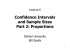### AP Statistics Name:_________________________________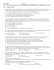### Statistical Reasoning for Everyday Life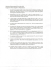### Sample size Student Learning Centre Semester 2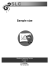### What is a sample proportion?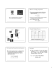### Document 6537306### MATH 140 SEMESTER REVIEW PROBLEMS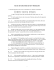### DETERMINING SAMPLE SIZE FOR RESEARCH ACTIVITIES ROBERT V. KREJCIE### measure attention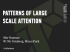### Statistics AP Chapter 10 Review: confidence interval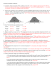### Ch7 Section 3: Confidence Intervals and Sample Size for Proportions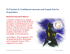### Lecture 3 Research Methods Lecture 10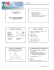### Proportion Power Point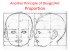### 1.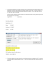### 3 clicks to total control – The new digital Grässlin time switch and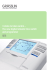### Confidence Intervals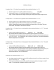### University of California, Davis Department of Statistics Summer Session II Statistics 13